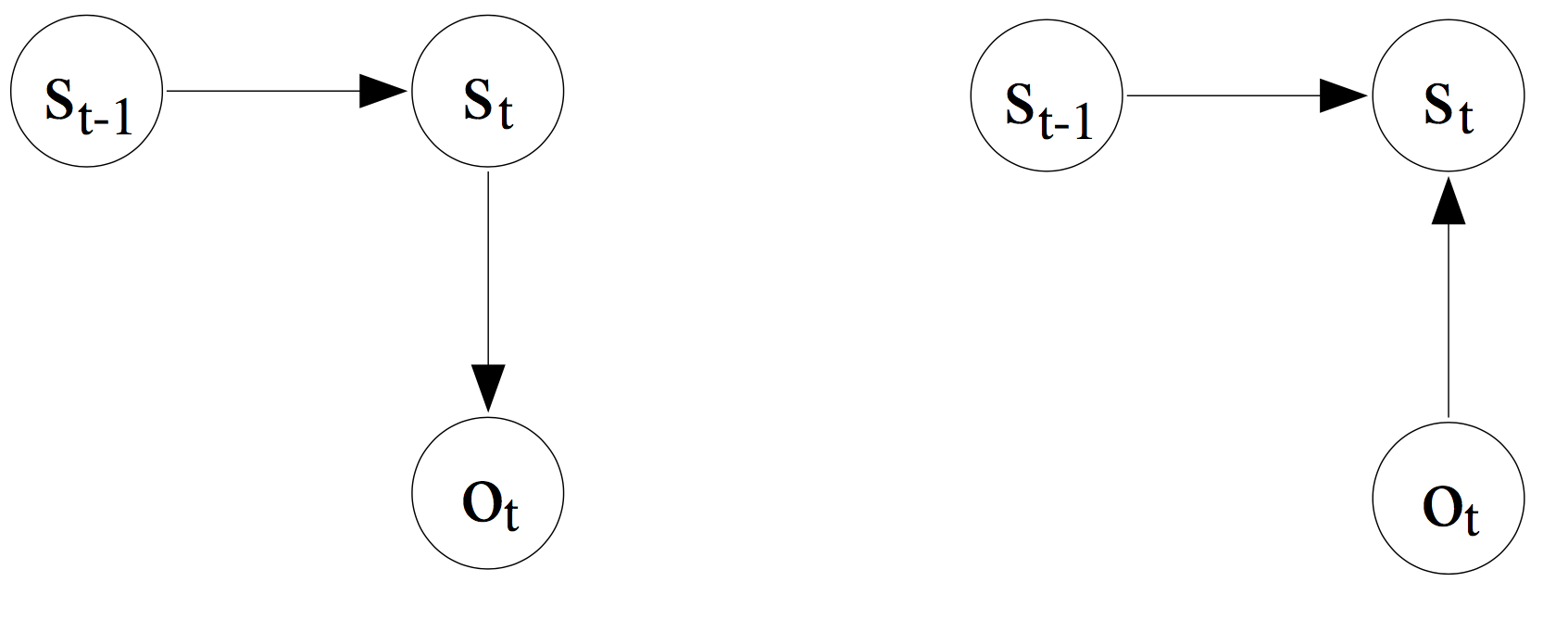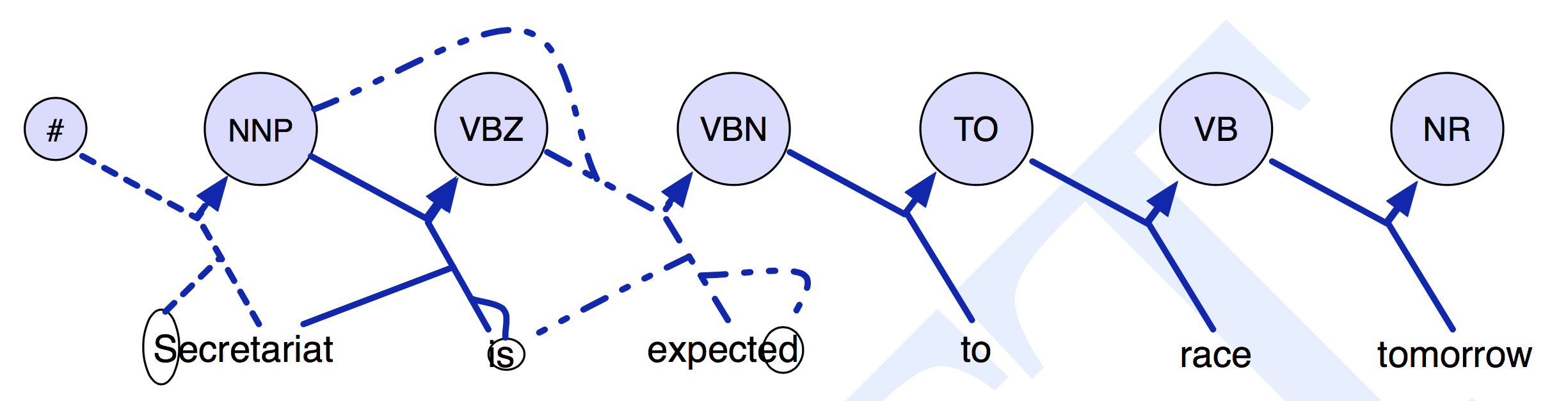This is the second part of a series of posts about sequential supervised learning applied to NLP. It can be seen as a follow up on the previous post, where I tried do explain the relationship between HMM and Naive Bayes. In this post I will try to explain how to build a sequence classifier based on a Logistic Regression classifier, i.e., using a discriminative approach.

You can find the first and third posts here:

## Discriminative vs. Generative Models

In a previous post I wrote about the Naive Bayes Model and how it is connected with the Hidden Markov Model. Both are generative models, in contrast, Logistic Regression is a discriminative model, this post will start, by explaining this difference.

In general a machine learning classifier chooses which output label $$y$$ to assign to an input $$x$$, by selecting from all the possible $$y_{i}$$ the one that maximizes $$P(y\mid x)$$.

The Naive Bayes classifier estimates $$p(y \mid x)$$ indirectly, by applying the Baye’s theorem, and then computing the class conditional distribution/likelihood $$P(x \mid y)$$ and the prior $$P(y)$$.

$\hat{y} = \underset{y}{\arg\max}\ P(y \mid x) = \underset{y}{\arg\max} \ P(x \mid y) \cdot P(y)$

This indirection makes Naive Bayes a generative model, a model that is trained to generated the data $$x$$ from the class $$y$$. The likelihood $$p(x \mid y)$$, means that we are given a class $$y$$ and will try to predict which features to see in the input $$x$$.

In contrast a discriminative model directly computes $$p(y \mid x)$$ by discriminating among the different possible values of the class $$y$$ instead of computing a likelihood. The Logistic Regression classifier is one of such type of classifiers.

$\hat{y} = \underset{y}{\arg\max} \ P(y \mid x)$

## Logistic Regression

Logistic regression is supervised machine learning algorithm used for classification, which is has it’s roots in linear regression.

When used to solve NLP tasks, it estimates $$p( y\mid x)$$ by extracting features from the input text and combining them linearly i.e., multiplying each feature by a weight and then adding them up, and then applying the exponential function to this linear combination:

$P(y|x) = \frac{1}{Z} \ \exp \sum_{i=1}^{N} w_{i} \cdot f_{i}$

where $$f_{i}$$ is a feature and $$w_{i}$$ the weight associated to the feature. The $$\exp$$ (i.e., exponential function) surrounding the weight-feature dot product ensures that all values are positive and the denominator $$Z$$ is needed to force all values into a valid probability where the sum is 1.

The extracted features, are binary-valued features, i.e., only takes the values 0 and 1, and are commonly called indicator functions. Each of these features is calculated by a function that is associated with the input $$x$$ and the class $$y$$. Each indicator function is represented as $$f_{i}(y,x)$$, the feature $$i$$ for class $$y$$, given observation $$x$$:

$P(y|x) = \frac{\exp \bigg( \sum\limits_{i=1}^{N} w_{i} \cdot f_{i}(x,y) \bigg)} {\sum\limits_{y' \in Y} \exp \bigg( \sum\limits_{i=1}^{N} w_{i} \cdot f_{i}(x,y') \bigg)}$

### Trainning

By training the logistic regression classifier we want to find the ideal weights for each feature, that is, the weights that will make training examples fit best the classes to which they belong.

Logistic regression is trained with conditional maximum likelihood estimation. This means that we will choose the parameters $$w$$ that maximize the probability of the $$y$$ labels in the training data given the observations $$x$$:

$\hat{w} = \underset{w}{\arg\max} \sum_{j} \log \ P(y^{j} \mid y^{j})$

The objective function to maximize is:

$L(w) = \sum_{j} \log\ P(y^{j} \mid y^{j})$

which by replacing with expanded form presented before and by applying the division log rules, takes the following form:

$L(w) = \sum\limits_{j} \log \exp \bigg( \sum\limits_{i=1}^{N} w_{i} \cdot f_{i} (x^{j},y^{j}) \bigg) - \sum\limits_{j} \log {\sum\limits_{y' \in Y} \exp \bigg( \sum\limits_{i=1}^{N} w_{i} \cdot f_{i}(x^{j},y'^{j}) \bigg)}$

Maximize this objective, i.e. finding the optimal weights, is typically solved by methods like stochastic gradient ascent, L-BFGS, or conjugate gradient.

### Classification

In classification, logistic regression chooses a class by computing the probability of a given observation belonging to each of all the possible classes, then we can choose the one that yields the maximum probability.

$\hat{y} = \underset{y \in Y} {\arg\max} \ P(y \mid x)$ $\hat{y} = \underset{y \in Y} {\arg\max} \frac{\exp \bigg( \sum\limits_{i=1}^{N} w_{i} \cdot f_{i}(x,y) \bigg)} {\sum\limits_{y' \in Y} \exp \bigg( \sum\limits_{i=1}^{N} w_{i} \cdot f_{i}(x,y') \bigg)}$

## Maximum Entropy Markov Model

The idea of the Maximum Entropy Markov Model (MEMM) is to make use of both the HMM framework to predict sequence labels given an observation sequence, but incorporating the multinomial Logistic Regression (aka Maximum Entropy), which gives freedom in the type and number of features one can extract from the observation sequence.

The HMM model is based on two probabilities:

• $$P( \text{tag} \mid \text{tag} )$$ state transition, probability of going from one state to another.

• $$P( \text{word} \mid \text{tag} )$$ emission probability, probability of a state emitting a word.

In real world problems we want to predict a tag/state given a word/observation. But, due to the Bayes theorem, that is, a generative approach, this is not possible to encode in the HMM, and the model estimates rather the probability of a state producing a certain word.

The MEMM was proposed as way to have richer set of observation features:

• “a representation that describes observations in terms of many overlapping features, such as capitalization, word endings, part-of-speech, formatting, position on the page, and node memberships in WordNet, in addition to the traditional word identity.”

and also to solve the prediction problem with a discriminative approach:

• “the traditional approach sets the HMM parameters to maximize the likelihood of the observation sequence; however, in most text applications […] the task is to predict the state sequence given the observation sequence. In other words, the traditional approach inappropriately uses a generative joint model in order to solve a conditional problem in which the observations are given.(Left) The dependency graph for a traditional HMM. (Right) The dependency graph for a Maximum Entropy Markov Model. (taken from A. McCallum et al. 2000)

In the Maximum Entropy Markov Models the transition and observation functions (i.e., the HMM matrices $$A$$ and $$B$$ from the previous post) are replaced by a single function:

$P(s_{t} \mid s_{t-1}, o_{t})$

the probability of the current state $$s_{t}$$ given the previous state $$s_{t-1}$$ and the current observation $$o$$. The figure below shows this difference in computing the state/label/tag transitions.Contrast in state transition estimation between an HMM and a MEMM. (taken from "Speech and Language Processing" Daniel Jurafsky & James H. Martin)

In contrast to HMMs, in which the current observation only depends on the current state, the current observation in an MEMM may also depend on the previous state. The HMM model includes distinct probability estimates for each transition and observation, while the MEMM gives one probability estimate per hidden state, which is the probability of the next tag given the previous tag and the observation.

In the MEMM instead of the transition and observation matrices, there is only one transition probability matrix. This matrix encapsulates all combinations of previous states $$S_{t−1}$$ and current observation $$O_{t}$$ pairs in the training data to the current state $$S_{t}$$.

Let $$N$$ be the number of unique states and $$M$$ the number of unique words, the matrix has the shape:

$(N \cdot M) \cdot N$

### Features Functions

The MEMM can condition on any useful feature of the input observation, in the HMM this wasn’t possible because the HMM is likelihood based, and hence we would have needed to compute the likelihood of each feature of the observation.

The use of state-observation transition functions, rather than the separate transition and observation functions as in HMMs, allows us to model transitions in terms of multiple, non-independent features of observations.

This is achieved by a multinomial logistic regression, to estimate the probability of each local tag given the previous tag (i.e., $$s'$$), the observed word (i.e. $$o$$), and any other features (i.e., $$f_{i}(x,y')$$) we want to include:

$P(s \mid s',o) = \frac{1}{Z(o,s')}\ \exp\bigg( \sum_{i=1}^{N} w_{i} \cdot f_{i}(o,s') \bigg)$

where, $$w_{i}$$ are the weights to be learned, associated to each feature $$f_{i}(o,s')$$ and $$Z$$ is the normalizing factor that makes the matrix sum to 1 across each row.Feature functions taking into consideration the whole observation sequence. (taken from "Speech and Language Processing" Daniel Jurafsky & James H. Martin)

### Training and Decoding

Taken from the original paper: “In what follows, we will split $$P(s \mid s', O)$$ into $$\mid S \mid$$ separately trained transition functions $$P_{s'} ( S \mid o) = P(s \mid s', O)$$. Each of these functions is given by an exponential model”

THE MEMM trains one logistic regression per state transition, normalised locally. The original MEMM paper, published in 2000, used a generalized iterative scaling (GIS) algorithm to fit the multinomial logistic regression, that is finding the perfect weights according to the training data. That algorithm has been largely surpassed by gradient-based methods such as L-BFGS.

For the decoding, the same algorithm as in the HMM is used, the Viterbi, although just slightly adapted to accommodate the new method of estimating state transitions.

### MEMM Important Observations

• The main advantage over the HMM is the use of feature vectors, making the transition probability sensitive to any word in the input sequence.

• There is an exponential model associate to each (state, word) pair to calculate the conditional probability of the next state.

• The exponential model allows the MEMMs to support long-distance interactions over the whole observation sequence together with the previous state, instead of two different probability distributions.

• MEMM can be also augmented to include features involving additional past states, instead of just the previous one.

• It also uses the Viterbi algorithm (slightly adapted) to perform decoding.

• It suffers from the label bias problem, I will detailed in the next post about Conditional Random Fields.

## Acknowledgments

The writing of this post is also the outcome of many discussions and white board sessions I had together with Tobias Sterbak